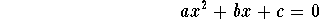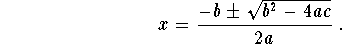PrevIndexNext

# Interpolation, String Operators, STDIN

## Interpolation

Variables occuring within a double quoted string are 'interpolated'.
\$x = 4; \$name = "Joe"; print "I am \$name. I am \$n years old.\n";
Very convenient.

Dollar Sign (\$) is special.
To get an actual dollar sign: \\$
To get an actual backslash: \\

SINGLE quoted strings take their contents literally - no interpolation, no backslash interpretation.

At Sign (@) is special, too - more on this later.

## Concatenation and Replication

There are two simple string operators: '.' and 'x' - dot/period and lower case x.
\$full = \$first . " " . \$last; # concatenated
Interpolation often works better than concatenation:
\$full = "\$first \$last";
The x operator replicates the string n times. It is not used often but is very convenient to have when you need it.
\$divider = "-" x 40; \$greet = "hello "; \$n = 5; print \$greet x \$n;

## Reading from the keyboard

Read from the keyboard with this construct:
print "Name? "; # note - no newline here \$name = <STDIN>;
STDIN must be capitalized.

This gets a line from the terminal keyboard up to a newline (Enter or Return). Note that the newline (\n) IS included at the end of the receiving variable.

print "Your name is \$name. Hello!\n";
This prints (Try it!) the following:
Your name is Joe . Hello!
This is not quite what you expect or want.

## Chopping and Chomping

The function 'chop' will remove the last character from \$name regardless of what it is.
chop \$name;
The kinder and gentler 'chomp' will remove one newline at the end of \$name. If the last character is not a newline it will have no effect at all.
chomp \$name;
Now we can rewrite the reading of the name from the keyboard like so:
print "Name? "; \$name = <STDIN>; chomp \$name; print "Your name is \$name. Hello!\n";

## Integer/String Conversion

Perl will convert between strings and numbers for you:
\$n = "34"; print \$n*3; # 102 \$n = 34; print "hello" . \$n; # hello34 print 123 x "3"; # 123123123
It will generally do what you want and expect it will do.

# Exercises

1. Redo the first exercise in the previous section using the new ideas presented here. Ask for the number rather than 'hard-coding' it in your program. Use string interpolation for output. Put a dividing line between the parts of the output.have two solutions which can be found with the quadratic formula:You may remember this from your days in high school algebra.

For example:

x**2 -5x + 6 = 0
This polynomial has two solutions: 2 and 3. Can you compute them by hand using the formula above? In this case a = 1, b = -5 and c = 6.

Perl has a square root function which takes one parameter:

\$x = sqrt 144; print "\$x\n"; # 12
Ask for values for a, b and c. Compute and then print out the two solutions. Note that some quadratic equations will have complex numbers as a solution (when the value (underneath the square root is negative). We'll deal with these soon. For now, just try these values for a, b, and c:
a 1 b 7 c 12 a 6 b -5 c -4